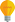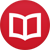# Derivative of $x^x$

by Jakub MarianTip: See my list of theMost Common Mistakes in English. It will teach you how to avoid mis­takes with com­mas, pre­pos­i­tions, ir­reg­u­lar verbs, and much more.

Calculating the derivative of $x^x$ is a very simple task, but it may be hard to find the general idea on your own, so here it is. We will need the following formula:

$$a^b = \l(e^{\ln(a)}\r)^b = e^{b\ln(a)}$$

(where “$\ln$” denotes the natural lnarithm, which is often denoted “$\ln$” in non-mathematical literature). The trick is to rewrite $x^x$ using the formula above (by setting $a = b = x$) and then differentiate the rest according to the normal rules of differentiation:

$$\l(x^x\r)’ = \l(e^{x\ln(x)}\r)’ = e^{x\ln(x)}\l(x\ln(x)\r)’\,,$$

where we used $(e^{f(x)})’ = e^{f(x)}f’(x)$. Now we will rewrite $e^{x\ln(x)}$ back to $x^x$ (it is the same thing we started with) and differentiate the second part using $(u(x)v(x))’ = u’(x)v(x) + u(x)v’(x)$:

$$e^{x\ln(x)}\l(x\ln(x)\r)’ = x^x\l(1⋅\ln(x) + x⋅\frac{1}{x}\r) = x^x\l(\ln(x)+1\r)\,.$$

And there you have it: $(x^x)’ = x^x\l(\ln(x)+1\r)$.

By the way, I have written several educational ebooks. If you get a copy, you can learn new things and support this website at the same time—why don’t you check them out?